### Efficiency of an IC Engine

The efficiency of an IC engine (Internal Combustion Engine) is defined as the ratio of workdone to the energy supplied to an engine. The following efficiencies of an 1.C. engine are important:

(a) Mechanical efficiency. It is the ratio of brake power (B.P.) to the indicated power (I.P.).

Mathematically, mechanical efficiency,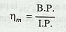Since B. P. is always less than I.P. , therefore mechanical efficiency is always less than unity (i.e. 100%).

(b) Overall efficiency. It is the ratio of the work obtained at the crankshaft in a given time to the energy supplied by the fuel during the same time. Mathematically, overall efficiency,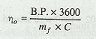where

B.P. = Brake power in kW,
mf = Mass of fuel consumed in kg per hour, and
C = Calorific valve of fuel in kJ / kg of fuel.

(c) Indicated thermal efficiency. It is the ratio of the heat equivalent to one kW hour to the heat in the fuel per I.P. hour, Mathematically, indicated thermal efficiency,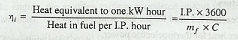Note : The following ratio is Known as specific fuel consumption per I.P. hour: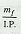(d) Brake thermal efficiency. It is the ratio of the heat equivalent to one kW hour to the heat in the fuel per B.P. hour. Mathematically, brake thermal efficiency,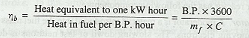Note: The following ratio is known as specific fuel consumption per B. P. hour: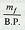(e) Air standard efficiency. The general expression for the air standard efficiency is given as

(For petrol engines)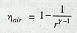(For diesel engines)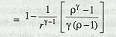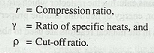(f) Relative efficiency. It is also known as efficiency ratio. The relative efficiency of an I. C. engine is the ratio of the indicated thermal efficiency to the air standard efficiency.

(g) Volumetric efficiency. It is the ratio of the actual volume of charge admitted during the suction stroke at N.T.P to the swept volume of the piston.

### Comments

1.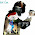2.very helpfull formulas

3.thank you... This helps me a lot